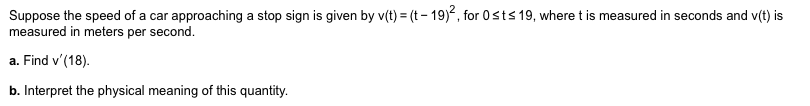# Suppose the speed of a car approaching a stop sign is given by v(t) (t-19)2, for 0sts 19, where t is measured in seconds and v(t) is measured in meters per second. a. Find v'(18) b. Interpret the physical meaning of this quantity.

Questionhelp_outlineImage TranscriptioncloseSuppose the speed of a car approaching a stop sign is given by v(t) (t-19)2, for 0sts 19, where t is measured in seconds and v(t) is measured in meters per second. a. Find v'(18) b. Interpret the physical meaning of this quantity. fullscreen